Courses

# Test: Spectroscopy Level - 3

## 30 Questions MCQ Test Organic Chemistry | Test: Spectroscopy Level - 3

Description
This mock test of Test: Spectroscopy Level - 3 for Chemistry helps you for every Chemistry entrance exam. This contains 30 Multiple Choice Questions for Chemistry Test: Spectroscopy Level - 3 (mcq) to study with solutions a complete question bank. The solved questions answers in this Test: Spectroscopy Level - 3 quiz give you a good mix of easy questions and tough questions. Chemistry students definitely take this Test: Spectroscopy Level - 3 exercise for a better result in the exam. You can find other Test: Spectroscopy Level - 3 extra questions, long questions & short questions for Chemistry on EduRev as well by searching above.
QUESTION: 1

Solution:
QUESTION: 2

Solution:
QUESTION: 3

### Which of the following compound show only two signals in 1H NMR and a strong IR bond at ~ 1690 cm-1:

Solution:
QUESTION: 4

The correct order of 1H NMR chemical shift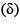values for the labeled methyl groups in the following compound is: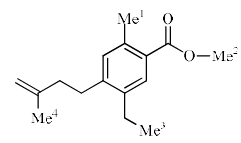Solution:
QUESTION: 5

The 1H NMR spectrum of a compound with molecular formula C3H7NO shows the following features: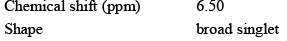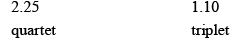Which of the following is in agreement with this information:

Solution:
QUESTION: 6

The two fine–structure components of a nuclear magnet ic resonance transit ion are observed at chemical shifts of 2.142 and 2.208 ppm in a 300 MHz NMR spectrometer. Calculate the coupling constant:

Solution:
QUESTION: 7

A C5H12O2 compound has strong infrared absorption at 3300 to 3400cm-1. The 1H NMR spectrum has three singlets at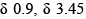and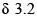ppm with relative areas 3:2:1. Addition of D2O to the sample eliminates the lower field signal. The 13C NMR spectrum shows three signals all at higher field than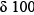ppm. Which of the following compounds best fits this data:

Solution:
QUESTION: 8

The 1H NMR spectrum of a compound A shows a doublet and a septet. Which one of the following statements is true:

Solution:
QUESTION: 9

A compound of formula C5H12 gives one signal in the 1H NMR and two signals in the 13C NMR spectra. The compound is:

Solution:
QUESTION: 10

The most appropriate spectroscopy for the identification of a nitrile group is:

Solution:
QUESTION: 11

Compound I gives a strong infrared absorption at 1730 cm-1. 1H NMR spectrum indicates that it has two types of hydrogen atoms; one H atom appearing as signlet at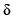= 9.7 ppm and 9H atoms appearing as a singlet at= 1.2 ppm. The structure of I is:

Solution:
QUESTION: 12

The functionality that shows a typical characteristic peak at 2250 cm-1 in IR is:

Solution:
QUESTION: 13

Consider a 1H NMR spectrum in which a quartet and a doublet appeared at 9.72 and 2.40 ppm, respectively. Which of the following compounds is the most probable one:

Solution:
QUESTION: 14

The order of decreasing chemicals shift in 1H NMR for the underlined hydrogens is:

(I) H3C – CH2 – CH3
(II) H3C – O – CH2 – CH3
(III) Cl2 – CH – O – CH2 – CH3
(IV) Cl – CH2 – O – CH2 – CH3

Solution:
QUESTION: 15

The number of normal modes of vibration in the benzene molecule is:

Solution:
QUESTION: 16

An organic compound (MF: C8H10O) exhibited the following 1H NMR spectral data:2.5 (3 H,s), 3.8 (3H, s), 6.8 (2 H, d, J 8 Hz), 7.2 (2 H, d, J 8 Hz) ppm. The compound among the choices is:

Solution:
QUESTION: 17

The 1H NMR spectrum of 1, 4-dimethoxybenzene will have:

Solution:
QUESTION: 18

Appropriate 1H NMR chemical shifts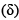for the protons A-D for the following compound are: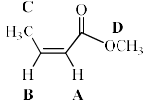Solution:
QUESTION: 19

Compound A and B exhibit two singlets, each in their 1H NMR spectra. The expected chemical shifts are at: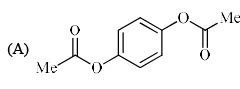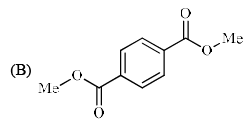Solution:
QUESTION: 20

In the 1H NMR spectrum recorded at 293 K, an organic compound (C3H7NO), exhibited signals at δ 7.8 (1H, s), 2.8 (3H, s) and 2.6 (3H, s). The compound is:

Solution:
QUESTION: 21

An organic compound exhibited the following 1H NMR spectra data:7.80 (2 H, d, J = 8 Hz), 6.80 (2 H, d, J = 8 Hz), 4.10 (2 H, q, J = 7.2 Hz), 2.4 (3H, s), 1.25 (3 H, t, J = 7.2 Hz)

The compound, among the choices given below is,

Solution:
QUESTION: 22

In NMR spectroscopy the product the nuclear ‘g’ factor (gN), the nuclear magneton (βN) and the magnetic field strength (B0) gives the:

Solution:
QUESTION: 23

Among the isomers of C4H6 given below, the compound which exhibits an absorption band at 3300 cm-1 in the IR spectrum, is:

Solution:
QUESTION: 24

Which of the following absorptions is shown by 1, 3-butadiene in its UV absorption spectrum recorded in n-hexane (εmax is the molar absorptivity):

Solution:
QUESTION: 25

Match the observed principal absorptions in the visible spectrum shown in List-I wit h the bond shows this absorption in List–II: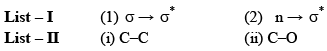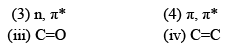Solution:
QUESTION: 26

The correct structure of the compound based on the following characteristic spectral data is IR: 1736 cm–1

1H NMR:3.59 (s, 3H), 3.32 (t, 2H), 2.25 (t, 2H), 1.85-1.75 (m, 2H), 1.73-1.62 (m, 2H)
13C NMR:174.0, 51.0M 32.9, 32.8, 31.0, 23.0

Solution:
QUESTION: 27

In atomic absorption spectroscopy, the atomization process utilizes:

Solution:
QUESTION: 28

The absorption at λmax 279 nm in the UV spectrum of acetone is due to:

Solution:
QUESTION: 29

An organic compound having the molecular formula C10H14 exhibited two singlets in the 1H NMR spectrum and three signals in the 13C NMR spectrum. The compound is:

Solution:
QUESTION: 30

A compound with molecular formula C5H10O, exhibit following 1H NMR spectral data -0.95 (6H, d),2.10 (3H, S),2.43 (1H, m). The structure is:

Solution: